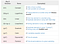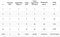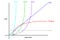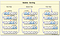# The Connection Between Time Complexity & Big O Notation Part 1

Understanding the time complexity of algorithms will help us assess if our code will scale. In implementing algorithms, it is also helpful to understand the variance in execution performance in order to select the best algorithm for your problem and achieve optimal efficiency.Source: https://img.devrant.com/devrant/rant/r_1204944_6PfxU.jpg

It’s important to note that time complexity is not the amount of measurable time (e.g., x number of seconds) that it takes for an algorithm to execute; rather it’s concerned with the number of operations that are performed. The number of operations executed by a program is affected by the size of input (referred to as n) and how their elements are arranged. To classify algorithms by their worst-case scenario, we use Big O Notation, which is a function of the input size n (e.g., O(n) or O(n³)). For concept visualization, a Big O cheatsheet, examples, and graph are listed below:Source: https://medium.com/@amejiarosario/8-time-complexity-examples-that-every-programmer-should-know-171bd21e5baSource: https://dzone.com/storage/temp/5068673-screen-shot-2017-04-24-at-21022-pm.pngSource: https://res.cloudinary.com/practicaldev/image/fetch/s--NR3M1nw8--/c_limit%2Cf_auto%2Cfl_progressive%2Cq_auto%2Cw_880/https://thepracticaldev.s3.amazonaws.com/i/z4bbf8o1ly77wmkjdgge.png

# 1. O(1): Constant Time

O(1) includes algorithms that take the same amount of time to execute regardless of the input size (n); these algorithms have a constant growth rate. Examples of O(1) runtime algorithms include:

`// 1. Checking if a number is even or odd`

In this example, it doesn’t matter if n is 1 or 1,000, it will execute the code block only one time.

`// 2. Printing the first element from a list`
`// 3. Checking if an item in an array is null`
`// 3. Finding a value on a hash map (which is generally done in O(1) time - in unique/difficult cases O(n) time)`

Note: It’s important to understand how operations (especially array and object methods) are being implemented in order to determine their runtime. For example, although the code block below is run only once per item — it’s actually run multiple times for each item in the array.

Additionally, believe it or not, primitive mathematical operations (e.g., +, *, -, /, and %) have a constant runtime as most programming languages limit numbers to a max value (in JavaScript, that Number.MAX_VALUE is 1. 7976…). Therefore, you cannot operate numbers that yield a greater value than the MAX_VALUE and these primitive mathematical operations are bound to be completed on a fixed amount of instructions (O(1)).

# 2. O(n): Linear Time

Algorithms that run in linear time are very common; in this runtime, it is implied that every element from the input is visited in the worst-case scenario. Linear time means that as the input grows, execution time grows proportionally. As the name suggests, this time complexity has a linear growth rate. Examples of O(n) runtime algorithms include:

`// 1. Finding the maximum and minimum values in an array`

The function getMaxValue() will check every element in the array. The counter was added to explicitly show how many times the function executed the code block. In this example, n == 4 (there are 4 items in the array) and the counter == 4 as well as soon as the execution was complete.

`// 2. Finding a given element in a collection`
`// 3. Printing all the values in a list`

# 3. O(n²) — Quadratic time

An algorithm with quadratic time complexity has a growth rate of n². For example, if the input (n) == 2, it will do 4 operations. Examples of O(n²) runtime algorithms include:

`// 1. Checking if a collection has any duplicate values`
`// 2. Sorting items in a collection using bubble sort, insertion sort, or selection sort`https://www.w3resource.com/w3r_images/bubble-short.png
`// 3. Finding all pairs in a collection`

# 4. O(n^c) — Polynomial time

In polynomial runtime, c > 1. O(n²) translates to two nested loops; three nested loops + are considered to be polynomial (cubic, quadratic, etc.). Polynomial runtimes take a great amount of time to compute as the input grows fast and therefore, are generally avoided. An example O(n^c) runtime algorithms includes:

`// 1. Finding the solution to a multi-variable equation (via triple nested loops)`

Graduate of Flatiron School’s Software Engineering Bootcamp as of 12/4/2020. Actively job searching in New York City.

## More from Madeline Stalter

Graduate of Flatiron School’s Software Engineering Bootcamp as of 12/4/2020. Actively job searching in New York City.